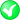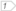#Boost C++ Libraries

...one of the most highly regarded and expertly designed C++ library projects in the world.

##### area (with strategy)

Calculates the area of a geometry using the specified strategy.

###### Description

The free function area calculates the area of a geometry using the specified strategy. Reasons to specify a strategy include: use another coordinate system for calculations; construct the strategy beforehand (e.g. with the radius of the Earth); select a strategy when there are more than one available for a calculation.

###### Synopsis

```template<typename Geometry, typename Strategy>
area_result<Geometry, Strategy>::type area(Geometry const & geometry, Strategy const & strategy)```

###### Parameters

Type

Concept

Name

Description

Geometry const &

Any type fulfilling a Geometry Concept

geometry

A model of the specified concept

Strategy const &

Any type fulfilling a Area Strategy Concept

strategy

The strategy which will be used for area calculations

###### Returns

The calculated area

Either

`#include <boost/geometry.hpp>`

Or

`#include <boost/geometry/algorithms/area.hpp>`

###### Conformance

The function area implements function Area from the OGC Simple Feature Specification.

###### Behavior

Case

Behavior

pointlike (e.g. point)

Returns 0

linear (e.g. linestring)

Returns 0

areal (e.g. polygon)

Returns the area

Cartesian

Returns the area in the same units as the input coordinates

Spherical

Returns the area on a unit sphere (or another sphere, if specified as such in the constructor of the strategy)

Reversed polygon (coordinates not according their orientiation)

Returns the negative area

###### Supported geometries

Geometry

Status

PointSegmentBoxLinestringRingPolygonMultiPointMultiLinestringMultiPolygonVariantLinear

###### Example

Calculate the area of a polygon

```#include <iostream>

#include <boost/geometry.hpp>
#include <boost/geometry/geometries/point_xy.hpp>
#include <boost/geometry/geometries/polygon.hpp>

namespace bg = boost::geometry;int main()
{
// Create spherical polygon
bg::model::polygon<bg::model::point<double, 2, bg::cs::spherical_equatorial<bg::degree> > > sph_poly;
bg::read_wkt("POLYGON((0 0,0 1,1 0,0 0))", sph_poly);

// Create spherical strategy with mean Earth radius in meters
bg::strategy::area::spherical<> sph_strategy(6371008.8);

// Calculate the area of a spherical polygon
double area = bg::area(sph_poly, sph_strategy);
std::cout << "Area: " << area << std::endl;

// Create geographic polygon
bg::model::polygon<bg::model::point<double, 2, bg::cs::geographic<bg::degree> > > geo_poly;
bg::read_wkt("POLYGON((0 0,0 1,1 0,0 0))", geo_poly);

// Create geographic strategy with WGS84 spheroid
bg::srs::spheroid<double> spheroid(6378137.0, 6356752.3142451793);
bg::strategy::area::geographic<> geo_strategy(spheroid);

// Calculate the area of a geographic polygon
area = bg::area(geo_poly, geo_strategy);
std::cout << "Area: " << area << std::endl;

return 0;
}
```

 Convenient namespace alias

Output:

```Area: 6.18249e+09
Area: 6.15479e+09
```